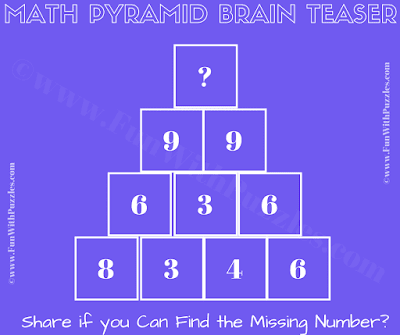## Saturday, December 21, 2019

This Tricky Math Riddle and Puzzle is for the higher school students who are expert in Maths. In this Math Pyramid Brain Teaser, there are numbers arranged in the Pyramid shape. Your challenge is to find the relationship between these numbers and then find the value of the missing number at the top of the pyramid. Let us see if you can solve this Tricky Math Riddle? Post your answers in the comment section once you are able to solve this Math puzzle.Can you solve this Math Riddle and Puzzle?

The answer to this "Maths Riddle and Puzzle", can be viewed by clicking on the answer button. Please do give your best try before looking at the answer.

1.wrong.
ans is 81
3*8 / 4 = 6, 3*4 / 4 = 3, 4*6 / 4 = 6
6*3 / 2 = 9, 3*6 / 2 = 9
9*9 / 1 = 81

1.Depending upon the solver's prospective, there could be many valid logical solutions to these puzzles.
Even though your and mine answers differ but both can be correctly obtained with different logical reasoning as explained by both us. So both the answers are correct.
Thanks for thinking differently and coming up with the different answer to this maths riddle.

A comment doesn't cost a thing. Please drop a comment below to boost the author's morale.